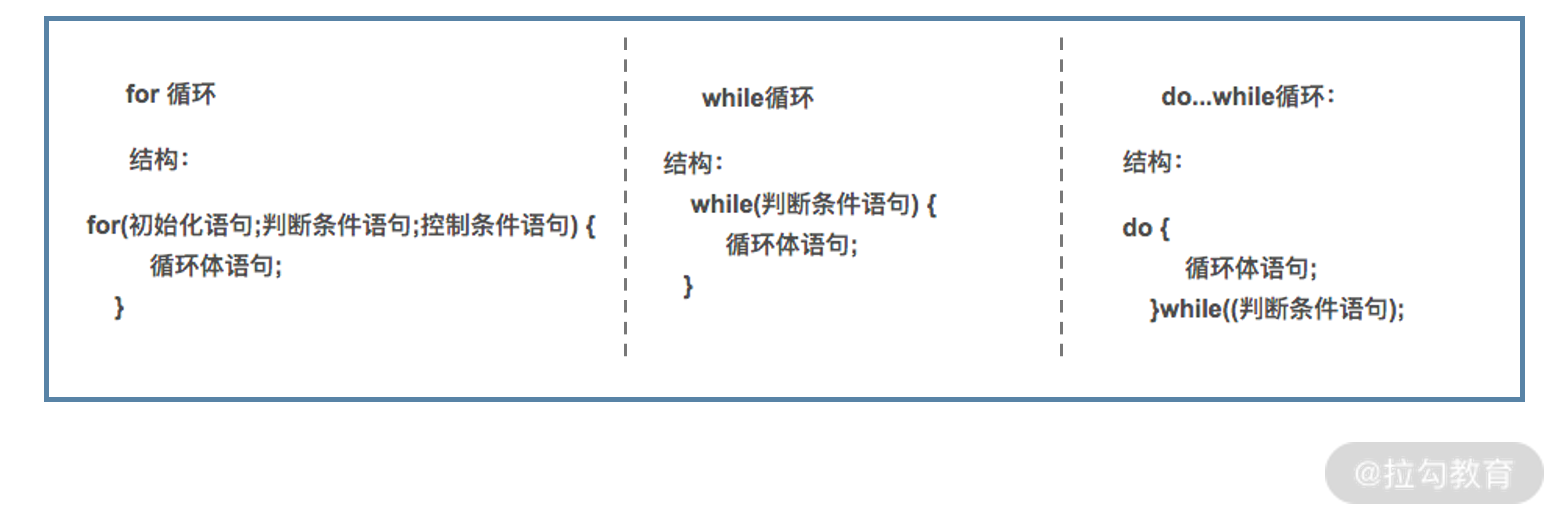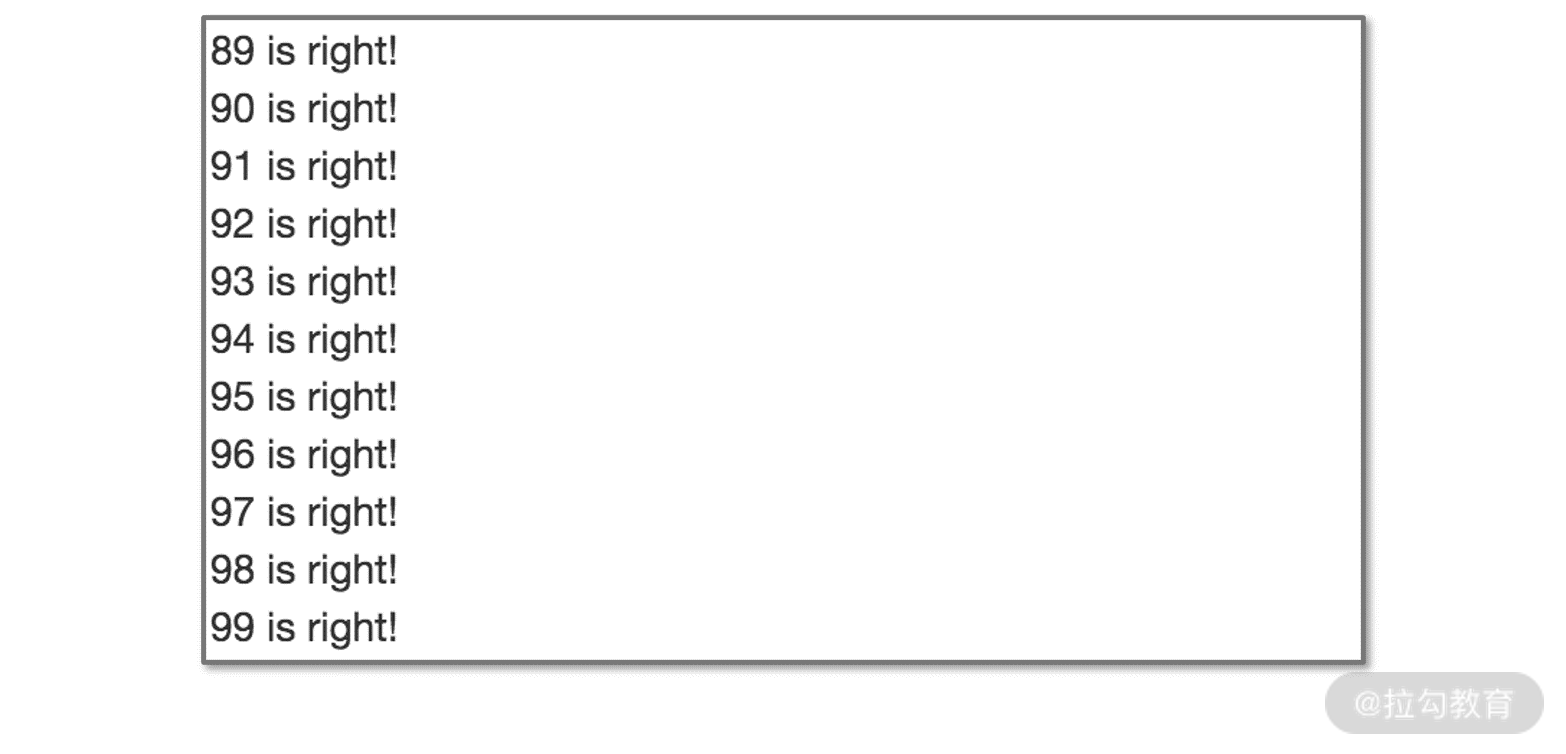14 程序的循环：如何利用数学归纳法进行程序开发？

从“多米诺骨牌”看循环归纳思想

• 第一，对于任意第 i 个骨牌而言，它的倒下能带动第 i+1 个骨牌倒下；
• 第二，有一个参与游戏的人手动推倒第一个骨牌。

“循环”的思想也存在我们的古文化中，《愚公移山》的“虽我之死，有子存焉。子又生孙，孙又生子；子又有子，子又有孙；子子孙孙无穷匮也。”简而言之就是，我有儿子，我儿子也有儿子，我儿子的儿子也会有儿子。以此类推，子子孙孙无穷尽。

• 第一，任意一代男子（或者说是儿子），都要再生至少一个儿子；
• 第二，愚公有个儿子。

数学归纳法

• 第一，当 n=1 时，命题成立；
• 第二，假设对于任意一个数字 i 命题成立，可以推导出在对于 i+1，命题依然成立。

【例 1】证明对于任意一个正整数 n，它的 2n 是偶数。

• 第一步，当 n=1 时，2n = 2×1 = 2 是偶数。
• 第二步，假设对于某个正整数 i 而言，2i 是偶数，则 2(i+1)=2i+2。其中 2i 为偶数，2 为偶数，两个偶数之和也是偶数，因此 2(i+1) 也是偶数。

【例 2】求证 1+3+5+...+(2k-1) = k2，我们依然可以用数学归纳法的思路来证明。

• 第一步，当 k=1 时，1=12 成立。
• 第二步，假设对于任意一个正整数 i 而言，1+3+5+...+(2i-1) = i2，则 1+3+5+...+(2i-1)+[2(i+1)-1] = i2+[2(i+1)-1] = i2+2i+2-1 = i2+2i+1 = (i+1)2 原命题依然成立。

循环结构

1.for 循环

for 循环的代码结构如下：

for(s1;s2;s3)

{

s4;

}

int result = 0;

for(int i= 1; i <= 50; i++)

{

result += i;

}

• 先执行 i=1，再判断 i≤50 与否，如果为真，则执行第 4 行的运算，最后执行 i++；
• 接着循环，再判断 i≤50 与否，如果为真，则执行第 4 行的运算，最后执行 i++；
• 经过多次循环后，再判断 i≤50 与否，直到结果为假，跳出循环结束。

for 循环还有很多变种，具体而言就是 s1、s2 和 s4 都可以被省略或部分省略。围绕上面的例子，s1 的定义可以单独抽出来放在第 2 行；而 for 循环语句中，可以空出 s1 的部分，这样新的代码可以写作：

int result = 0;

int i= 1;

for(; i <= 50; i++)

{

result += i;

}

int result = 0;

int i= 1;

for(; i <= 50; )

{

result += i;

i++;

}

int result = 0;

int i= 1;

for(; ; )

{

if (i > 50){

break;

}

result += i;

i++;

}

2.while 循环

while (s2)

{

s4;

}

while 循环的执行顺序是 (s2,s4)、(s2,s4)...(s2,s4)、s2。具体而言，是首先判断 s2 是否成立，如果为真，则执行 s4；继续循环判断 s2 是否成立，如果为真，则执行 s4；如此循环多次后，直到 s2 不再成立，跳出循环结束。

int i = 0;

int result = 0;

while (i < =50)

{

result += i;

}

int i = 0;

int result = 0;

while (1)

{

if (i > 50){

break;

}

result += i;

}

3.do while 循环

do {

s4;

}while(s2);

do while 循环与 while 循环相比，区别就是执行顺序的调整。do while 循环中，无论 s2 是真是假，都会至少执行一次 s4。这样它的执行顺序就是 (s4,s2)、(s4,s2)...(s4,s2)。

int i = 1;

int result = 0;

do {

result += i;

}while(i <= 49);

do while 循环也有一些变种，其 s2 语句也可以被调整到其循环体中，可以考虑用 if 条件语句和 break 语句实现：

int i = 1;

int result = 0;

do {

result += i;

if (i > 49){

break;

}

}while(1);

4.三种循环结构的区别for(s1;s2;s3)

{

s4;

}

s1;

while(s2)

{

s4;

s3;

}

s1;

do {

if(!s2)

{

break;

}

s4;

s3;

}while(1);

数学归纳法与循环结构

• 数学归纳法不关注归纳过程的结束，它就是用一种重复动作，由有穷尽朝着无穷尽的方向去前进；
• 而循环结构作为一种程序开发逻辑，则必须要关注循环过程的结束，否则就会造成系统陷入死循环或死机。

• 证明 k=1 时等式成立；
• 假设 k=i 时等式成立后，k=i+1 等式依然成立。

• 在这个框架中，最开始的 s1、s2、s4，即为当 k=1 时等式成立，对应数学归纳法的第一步。
• 在这个框架中，任意相邻的两组(s2,s4,s3)、(s2,s4,s3)，就是假设 k=i 时等式成立后，k=i+1 等式依然成立，对应数学归纳法的第二步。

int left = 0;

int left_temp = 0;

int right = 0;

for (int k = 1; k < 100; k++) // s1;s2;s3

{

//s4

left_temp = 2 * k - 1;

left += left_temp;

right = k * k;

if (left == right)

{

printf("%d is right!\n",k);

}

}

• 代码的前三行定义了 3 个变量，分别是 left、left_temp 和 right，其中 left 和 right 分别用来存储等式两边的结果，left_temp 用来存储公式中每轮增加的一项；
• 第 4 行，进入 for 循环，得到对应的 s1、s2 和 s3；
• 第 6 行，计算出当前一轮的 left_temp 值；
• 第 7 行，把 left_temp 作为增量，增加到 left 的值中；
• 第 8 行，计算等式右侧的 k2 的值；
• 第 9 行，对等式左边和等式右边是否相等做出判断；
• 第 10～12 行进行判断，如果等式相等，打印结果，代码的部分执行结果如下图。